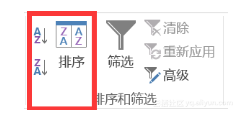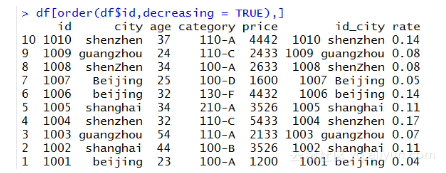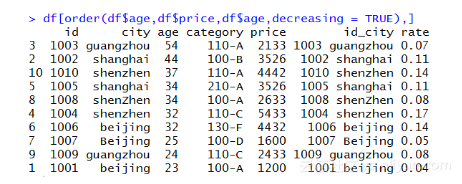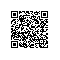# 《从Excel到R 数据分析进阶指南》一4.2 数据排序

### 4.2 数据排序

Excel中可以通过数据目录下的排序按钮直接对数据表进行排序，比较简单。R中需要使用order()函数完成排序。#对数据表按id列进行排序(逆序)
df[order(df$id,decreasing = TRUE),]省略decreasing参数默认为正序排序。下面的代码按rate对数据表进行排序。 #对数据表按rate列进行排序(正序) df[order(df$rate),]

#对多列进行排序
df[order(df$age,df$price,df\$age,decreasing = TRUE),]使用钉钉扫一扫加入圈子
+ 订阅